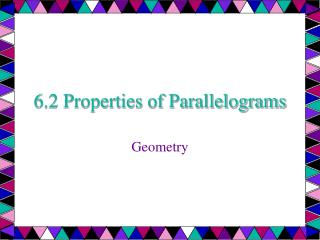DownloadDownload Presentation6.2 Properties of Parallelograms

# 6.2 Properties of Parallelograms

Download Presentation## 6.2 Properties of Parallelograms

- - - - - - - - - - - - - - - - - - - - - - - - - - - E N D - - - - - - - - - - - - - - - - - - - - - - - - - - -
##### Presentation Transcript

1. 6.2 Properties of Parallelograms Geometry

2. Objectives: • Use properties of parallelograms.

3. In this lesson . . . A parallelogram is a quadrilateral with both pairs of opposite sides parallel. When you mark diagrams of quadrilaterals, use matching arrowheads to indicate which sides are parallel. For example, in the diagram to the right, PQ║RS and QR║SP. The symbol PQRS is read “parallelogram PQRS.”

4. If a quadrilateral is a parallelogram, then its opposite sides are congruent. ►PQ≅RS and SP≅QR Theorems about parallelograms Q R P S

5. If a quadrilateral is a parallelogram, then its opposite angles are congruent. P ≅ R and Q ≅ S Theorems about parallelograms Q R P S

6. If a quadrilateral is a parallelogram, then its consecutive angles are supplementary (add up to 180°). mP +mQ = 180°, mQ +mR = 180°, mR + mS = 180°, mS + mP = 180° Theorems about parallelograms Q R P S

7. If a quadrilateral is a parallelogram, then its diagonals bisect each other. QM ≅ SM and PM ≅ RM Theorems about parallelograms Q R M • Remember BISECT means to cut in half EQUALLY! P S

8. FGHJ is a parallelogram. Find the unknown length. Explain your reasoning. JH JK Ex. 1: Using properties of Parallelograms 5 G F 3 K H J b.

9. FGHJ is a parallelogram. Find the unknown length. Explain your reasoning. JH JK SOLUTION: a. JH = FG Opposite sides of a are ≅. JH = 5 Substitute 5 for FG. Ex. 1: Using properties of Parallelograms 5 G F 3 K H J b.

10. FGHJ is a parallelogram. Find the unknown length. Explain your reasoning. JH JK SOLUTION: a. JH = FG Opposite sides of a are ≅. JH = 5 Substitute 5 for FG. Ex. 1: Using properties of Parallelograms 5 G F 3 K H J • JK = GKDiagonals of a bisect each other. • JK = 3 Substitute 3 for GK b.

11. PQRS is a parallelogram. Find the angle measure. mR mQ Ex. 2: Using properties of parallelograms Q R 70° P S

12. PQRS is a parallelogram. Find the angle measure. mR mQ a. mR = mP Opposite angles of a are ≅. mR = 70° Substitute 70° for mP. Ex. 2: Using properties of parallelograms Q R 70° P S

13. PQRS is a parallelogram. Find the angle measure. mR mQ a. mR = mP Opposite angles of a are ≅. mR = 70° Substitute 70° for mP. mQ + mP = 180° Consecutive s of a are supplementary. mQ + 70° = 180° Substitute 70° for mP. mQ = 110° Subtract 70° from each side. Ex. 2: Using properties of parallelograms Q R 70° P S